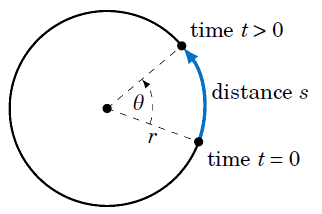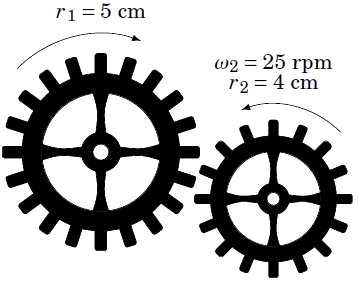# Circular Motion: Linear and Angular Speed

### Article objectives

• To calculate the speed and angular velocity of objects.
• To understand the relationship between linear and angular speed.
• Radian measure and arc length can be applied to the study of circular motion. In physics the average speed of an object is defined as:

$$\text{average speed} = \frac{\text{distance traveled}}{\text{time elapsed}}$$So suppose that an object moves along a circle of radius r, traveling a distance s over a period of time t, as in Figure 1. Then it makes sense to define the (average) linear speed ν of the object as:

$$v = \frac{s}{t} \; \; \; \; (1)$$

Let θ be the angle swept out by the object in that period of time. Then we define the (average) angular speed ω of the object as:

$$ω = \frac{θ}{t} \; \; \; \; (2)$$

Angular speed gives the rate at which the central angle swept out by the object changes as the object moves around the circle, and it is thus measured in radians per unit time. Linear speed is measured in distance units per unit time (e.g. feet per second). The word linear is used because straightening out the arc traveled by the object along the circle results in a line of the same length, so that the usual definition of speed as distance over time can be used. We will usually omit the word average when discussing linear and angular speed here.

Since the length s of the arc cut off by a central angle θ in a circle of radius r is s = r θ, we see that

$$v = \frac{s}{t} = \frac{r θ}{t} = \frac{θ}{t} . r$$

so that we get the following relation between linear and angular speed:

$$v = ω r \; \; \; \; (3)$$

Example 1

An object sweeps out a central angle of $$\frac{π}{3}$$ radians in 0.5 seconds as it moves along a circle of radius 3m. Find its linear and angular speed over that time period.

Solution: Here we have t = 0.5 sec, r = 3 m, and θ = $$\frac{π}{3}$$ rad. So the angular speed ω is

$$ω = \frac{θ}{t} = \frac{\frac{π}{3} rad}{0.5 sec} ⇒ ω = \boxed{\frac{2π}{3} rad/sec},$$

and thus the linear speed ν is

$$v = ω r = \left(\frac{2π}{3} rad/sec \right) (3 m) ⇒ \boxed{v = 2π m/sec}$$

Note that the units for ω are rad/sec and the units of ν are m/sec. Recall that radians are actually unitless, which is why in the formula ν = ωr the radian units disappear.

Example 2

An object travels a distance of 35 ft in 2.7 seconds as it moves along a circle of radius 2 ft. Find its linear and angular speed over that time period.

Solution: Here we have t = 2.7 sec, r = 2 ft, and s = 35 ft. So the linear speed ν is

$$v = \frac{s}{t} = \frac{35 feet}{2.7 sec} ⇒ \boxed{12.96 ft/sec} ,$$

and thus the angular speed ω is given by

$$v = ω r = 12.96 ft/sec = ω(2 ft) ⇒ \boxed{ω = 6.48 rad/sec}$$

Example 3

An object moves at a constant linear speed of 10 m/sec around a circle of radius 4 m. How large of a central angle does it sweep out in 3.1 seconds?

Solution: Here we have t = 3.1 sec, ν = 10 m/sec, and r = 4 m. Thus, the angle θ is given by

$$s = rθ ⇒ θ = \frac{s}{r} = \frac{vt}{r} = \frac{(10 m/sec)(3.1 sec)}{4 m} = \boxed{7.75 rad}.$$

In many physical applications angular speed is given in revolutions per minute, abbreviated as rpm. To convert from rpm to, say, radians per second, notice that since there are 2π radians in one revolution and 60 seconds in one minute, we can convert N rpm to radians per second by “canceling the units” as follows:

$$N rpm = N \frac{rev}{min} . \frac{2π}{1 rev} . \frac{1 min}{60 sec} = \frac{N . 2π}{60} rad/sec$$

This works because all we did was multiply by 1 twice. Converting to other units for angular speed works in a similar way. Going in the opposite direction, say, from rad/sec to rpm, gives:

$$N rad/sec = \frac{N . 60}{2π} rpm$$

Example 4

A gear with an outer radius of r1 = 5 cm moves in the clockwise direction, causing an interlocking gear with an outer radius of r2 = 4 cm to move in the counterclockwise direction at an angular speed of ω2 = 25 rpm. What is the angular speed ω1 of the larger gear?Solution: Imagine a particle on the outer radius of each gear. After the gears have rotated for a period of time t > 0, the circular displacement of each particle will be the same. In other words, s1 = s2, where s1 and s2 are the distances traveled by the particles on the gears with radii r1 and r2, respectively. But s1 = ν1 t and s2 = ν2 t, where ν1 and ν2 are the linear speeds of the gears with radii r1 and r2, respectively. Thus,

$$v_{1}t = v_{2}t ⇒ v_{1} = v_{2}$$

so by formula (3) we get the fundamental relation between the two gears:

$$\boxed{ω_{1}r_{1} = ω_{2}r_{2}}$$

Note that this holds for any two gears. So in our case, we have

$$ω_{1}(5) = (25)(4) ⇒ \boxed{ω_{1} = 20 rpm} .$$